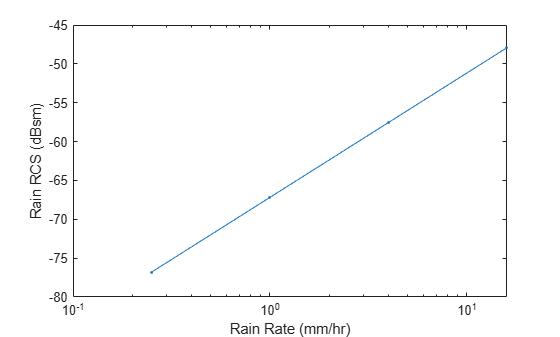# clutterVolumeRCS

## Syntax

``rcs = clutterVolumeRCS(volrefl,vol)``

## Description

example

````rcs = clutterVolumeRCS(volrefl,vol)` returns the radar cross-section (RCS) of volume clutter defined by the resolution of the radar.```

## Examples

collapse all

Estimate the radar cross-section of rain for a side-looking airborne SAR operating in the L band at 1.5 GHz. The rain is specified by a range resolution of 15 m, an azimuth resolution of 18 m, and a rain elevation cell resolution of 20 m. The rain rates are 0.25 mm/hr, 1 mm/hr, 4 mm/hr, and 16 mm/hr.

```f = 1.5e9; rngres = 15; azres = 18; elres = 20; res = [rngres azres elres]; rr = [0.25 1 4 16];```

Compute the rain radar cross-section. Use `rainreflectivity` to compute the volume reflectivity of the scattering particles.

```volref = rainreflectivity(f,rr); rcs = clutterVolumeRCS(volref,res);```

Plot the rain radar cross-section as a function of the rain rate. Express the cross-section in dB.

```semilogx(rr,pow2db(rcs),'.-') xlabel('Rain Rate (mm/hr)') ylabel('Rain RCS (dBsm)')```## Input Arguments

collapse all

Volume reflectivity of scattering particles in square meters per cubic meter, specified as a scalar or a vector.

Data Types: `double`

Clutter extent, specified as a positive real scalar or a 1-by-3 row vector.

• If specified as a positive real scalar, `vol` represents the volume of the clutter in cubic meters.

• If specified as a 1-by-3 row vector:

• The first element of `vol` is a positive real scalar that represents the clutter within the range resolution in meters of the radar.

• The second element of `vol` is a positive real scalar that represents the clutter within the azimuth (or cross-range) resolution in meters of the radar.

• The third element of `vol` is a positive real scalar that represents the clutter within the elevation resolution in meters of the radar.

Data Types: `double`

## Output Arguments

collapse all

Radar cross-section of volume clutter in square meters, returned as a scalar or a vector. `rcs` has the same dimensions as `volrefl`.

## Version History

Introduced in R2021a